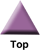### Home

Laboratory
Studies

Recordkeeping, Writing,
& Data Analysis

Laboratory
Methods

Overview
Microscope studies

Flagella experiment
Laboratory math
Blood fractionation
Gel electrophoresis
Protein gel analysis
Mitochondria
Concepts/ theory
Overview
Keeping a lab notebook
Writing research papers
Dimensions & units
Using figures (graphs)
Examples of graphs
Experimental error
Representing error
Applying statistics
Overview
Principles of microscopy

Solutions & dilutions
Protein assays
Spectrophotometry
Fractionation & centrifugation

# Making Dilutions

Many of you appear to panic when you must dilute something, yet the mathematics involve nothing worse than the simplest algebra. One reason is simply that when you are busy with a laboratory procedure you are distracted and it is difficult to think in the abstract. That problem can be overcome by practicing in advance of the need. Even with practice, though, you may find dilution problems confusing unless you very clearly define your objectives. We will give you a useful formula for making dilutions, one that you may have seen before. The formula is worse than useless, though, if you don't use it properly.

## Notes on using micropipettors

• To obtain the best accuracy with variable volume pipettors pre-rinse each new disposable tip
• To avoid error due to hysteresis when setting volume on a variable volume pipettor be consistent in the direction in which you change volume (either always increase to the desired volume or always decrease to the desired volume)
• When conducting a dilution using a micropipettor make sure that the tip can reach the bottom of the test tube; for example, our 1000 µl pipettors with blue tips cannot reach the bottom of a 13 x 100 mm culture tube; use an Eppendorf sample tube instead
• It is very awkward to have one person hold a tube while the other pipets from it; when students work in pairs it is better simply to take turns pipetting

## Establish a frame of reference

For the sake of simplicity, lets say we are talking about sucrose solutions. Suppose you have a starting solution of sucrose (in water) with volume V1 and concentration C1. What is the total amount of sucrose in your solution? Answer: C1•V1.

Example. Volume = 0.2 liter; concentration is 50 grams/liter. C1•V1 = 50 grams/liter • 0.2 liter= 10 grams sucrose.

Now suppose that you dilute that solution with water—the whole thing—to some larger, predetermined volume (V2). What amount of sucrose is present in the new, diluted solution? If you said 10 grams, you get the gold star. But wait a minute—C1•V1 = 10 grams, and the new solution has a different volume, V2. The same amount of sucrose is present in the new solution as was in the original solution, so the following relationship must hold:

C1*V1 = C2*V2, where C2 = concentration of the new solution.

Example. Dilute the previous sucrose solution to 2 liters. What is the concentration of the new solution? We must solve for C2, of course. C1•V1 = C2•V2 = 10 grams. We know that V2 = 2 liters, so now we have

C2•(2 liters) = 10 grams

Solve for C2 to obtain 5 grams/liter.

Determining what you already knows and putting the informatin into the equation C1•V1 = C2•V2 establishes the relationship that you need in order to solve dilution problems.

## Determine the objective

What do you want to do? Or, more realistically, what does the instructor want you to do? Two types of diution problems are quite common in biology and biochemistry labs.

• Dilute a known volume of known concentration to a desired final concentration
• Dilute a known concentration to a desired final concentration AND volume

The second type of problem really throws people off! Let's start with the first one, though. You know V1, C1, and C2 is predetermined. It remains, then, to solve for V2, namely the final volume to which to dilute the solution. This one is easy, since you keep the amount of solute the same and only have to change one factor.

Now for the second type problem. You know C1, namely the concentration of the starting solution. You have predetermined both V2 and C2, namely the final volume and concentration that you desire. There is one undetermined variable left, namely V1. V1 is the volume of original solution that you will dilute to the desired final volume and concentration.

C1•V1 = C2•V2

V1 = (C2•V2)/C1 or,

V1 = (final amount of solute)/C1

Example. You have a sucrose solution of 47 grams/liter. You want to prepare 100 milliliters (0.1 liter) of sucrose solution of concentration 25 grams/liter. Since you know the starting concentration of sucrose and you know both the final concentration and volume of solution that you want, all you need to find out is what volume of starting solution (V1) to use.

V1 = (C2•V2)/C1, that is, V1 = (25 grams/liter•0.1 liter) ÷ 47 grams/liter = 0.053 liter = 53 ml

Notice that the calculation comes out to 0.05319149L using full precision, but I rounded off the required volume to the nearest ml. There is a limit to the precision with which we can prepare a solution, and also a limit to the precision that we really need. You would use a 100 ml graduated cylinder to determine final volume. You would be able to read the markings to the nearest 1 ml, as a rule.

## Units

It is critical that you report units for concentrations, volumes, and amounts, and when you make calculations for dilutions you must not mix up the units. For example, it doesn't work to write,

V1 (160 milliters)•C1(160 milligrams/liter = V2 (unknown)•C2(desired-3 grams/liter)

However, because concentration represents a proportional relationship, you can select from a variety of units. For example, 1 milligram/milliliter is the same as 1 gram/liter, 1 microgram/microliter, or 1 nanogram/nanoliter. It is also the same as 1000 milligrams/liter, but why would we write it that way?

Select units that simplify your expressions.